# Gyrogravity and Antigravity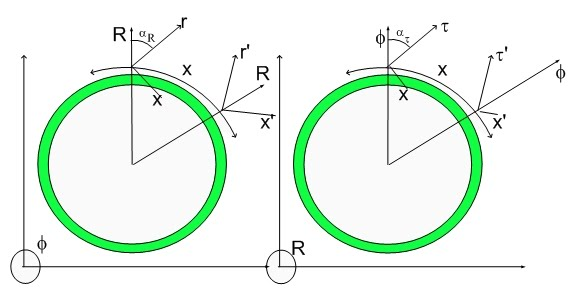Gyrogravitation and AntiGravity

When I replicated Newton's Law of Gravitation using my model - which is more fundamental than the current perception of Nature - I was happy. Now that I improved upon Newton's understanding of Gravitation, I am ecstasic.

This is a very important blog..:) The new understanding was derived using the same reasoning I used to derive Biot-Savart Law. As you know, Biot-Savart Law describes the forces among charged particles in motion. I derived the same law for non-charged particles in motion or the Hypergeometrical Law of Gravitation.

It points towards the existence of Antigravity right there... in Gravity...:) where you least expected..>:)

It also points to the demise of the paradigm Einstein made popular. The one where mass just deforms spacetime...:) Now that model would have to include quite a bit of complication due to the velocity dependent spacetime deformations. I believe that would be unnecessary and obsolete due to my theory. It doesn't provide such a beautiful picture as it did just a second ago..>:)

Much has been said about antigravity and its role in the Universe expansion. Of course, there is no need for antigravitational forces for the expansion of the Universe given my proposed Hyperspherical Lightspeed Expanding Shockwave Universe.

Despite of that, there is such a thing as an antigravitational component to Gravity. Below is the derivation also shown in my papers.

3.3 GyroGravitation

Similarly one can derive the Gravitational Biot-Savart equation by simple analogy to our derivation of the Gravitation Law.

Equation 3.41 providing the displacement has to be modified for attraction between spin zero dilators and simplified: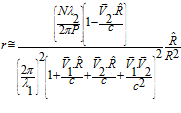(3.41)

The limit with zero velocity independent term corresponds to the steady state gravitational field (Newton's Law).

Of course, any change in the formula for gravitation to include velocity dependent terms would deem General Gravitation paradigm incorrect and unworkable. The paradigm I am addressing is the deformation of spacetime by mass distribution.

Again, the total gravitational force between two 1 kg test Mass is given by: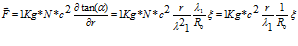We obtain: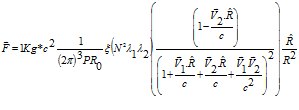Using equation (3.25)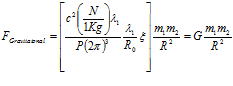We obtained the correct Law of Gravitation: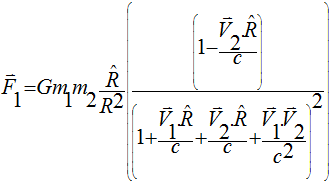(3.49b)

or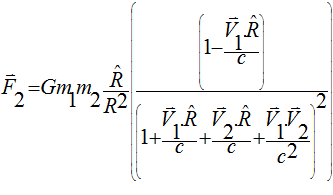(3.49c)

From equation (3.26) it is clear that R points from position 1 to position 2, F1 is force acting upon body 1 under the influence of body 2. There is an inherent asymmetry due to the usage of a third inertial reference frame which is inertial. Any reference frame based upon either body would be non-inertial. This equation was derived under the regimen of weak (normal) gravitational pull. It would be easy to derived the same equation for conditions in the surroundings of a Black Hole. One would just not use the derivative approximations.

This means that there is AntiGravity right within the Law of Gravitation. If for a moment one sets the referential frame on body 1, thus having V1=0, the Gravitational Force on F2 becomes: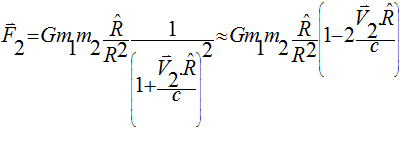(3.49d)

That is, the force decreases as the velocity increases, thus Gravitation produces an outwards acceleration. This is what one would expect from Antigravity.

This is a much more complex view of Gravitation and it is the only view derived from a more fundamental model. It reduces to Newton's Law at zero relative velocity.

This equation is likely to explain Pioneer anomaly, jets emanating from Black Holes since it produces an element of antigravity which depends upon the state of motion of the Black Hole or the Sun in the case of the Pioneer anomaly.

This is a much more complex view of Gravitation and it is the only view derived from a more fundamental model. It reduces to Newton's Law at zero relative velocity.

The second term in equation (3.49d) corresponds to the Antigravitational Force. It negative sign means that it is a repulsion or lessening of attraction.

Pioneer anomaly and Black Hole emanating jets are easily explainable using this equation. A non-approximated equation is easily derived following the ideas in my paper. Integration of this law within an stellar body will bring about a much more complex interaction that the one expected from Newton's Law of Gravitation.

Cheers,

MP

PS-  I polished the pdf a little bit.  I tracked down two (1Kg) factors hidden in the definition of epsilon zero and mu zero (vacuum magnetic susceptibility and vacuum electric permittivity).
Without them the formulas for epsilon zero and mu zero seem to have the incorrect units.  I also made the wavevectors to be (1x5) vectors instead of (5x1), otherwise it seems that I don't know how to make a matrix multiplication..:)

I took the initiative to send an email to the good Nasa folks behind the Pioneer Anomaly paper.  I don't have the time nor the data to use this equation on planetary dynamics or Pioneer anomaly.

The other great news is that I was able to engage Ernest S. Gullible (not his real name) to be kind and take a view on my theory and provide some fierce critique.   Despite of this name, the man is known or should be well known for being someone with a keen eye for crappy science...:)

If he provides any critique I will be happy to post here and correct anything he might find incorrect.:)
Currently unrated

### New Comment

required

required (not published)

optional

required